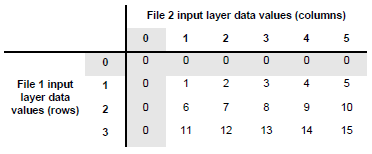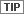# Matrix Analysis

## Producer Field Guide

HGD_Product
Producer Field Guide
HGD_Portfolio_Suite
Producer

Matrix analysis produces a thematic layer that contains a separate class for every coincidence of classes in two layers. The output is best described with a matrix diagram.In this diagram, the classes of the two input layers represent the rows and columns of the matrix. The output classes are assigned according to the coincidence of any two input classes. Unlike overlaying or indexing, the resulting class values of a matrix operation are unique for each coincidence of two input class values.All combinations of 0 and any other class are coded to 0, because 0 is usually the background class, representing an area that is not being studied.

In this example, File 1 may define class 1 as water and class 3 as soil while File 2 may define class 1 as warm and class 3 as cool. The output class value at column 1, row 3 is 11 which would correspond to warm soil, and the output class at column 3, row 1 is 3 which would correspond to cool water. These are clearly two entirely different classes. If these files had been indexed (summed) instead of matrixed, both combinations would be coded to class 4.

Use the Matrix (GIS Analysis) function in the IMAGINE Workspace or Spatial Modeler to matrix layers.